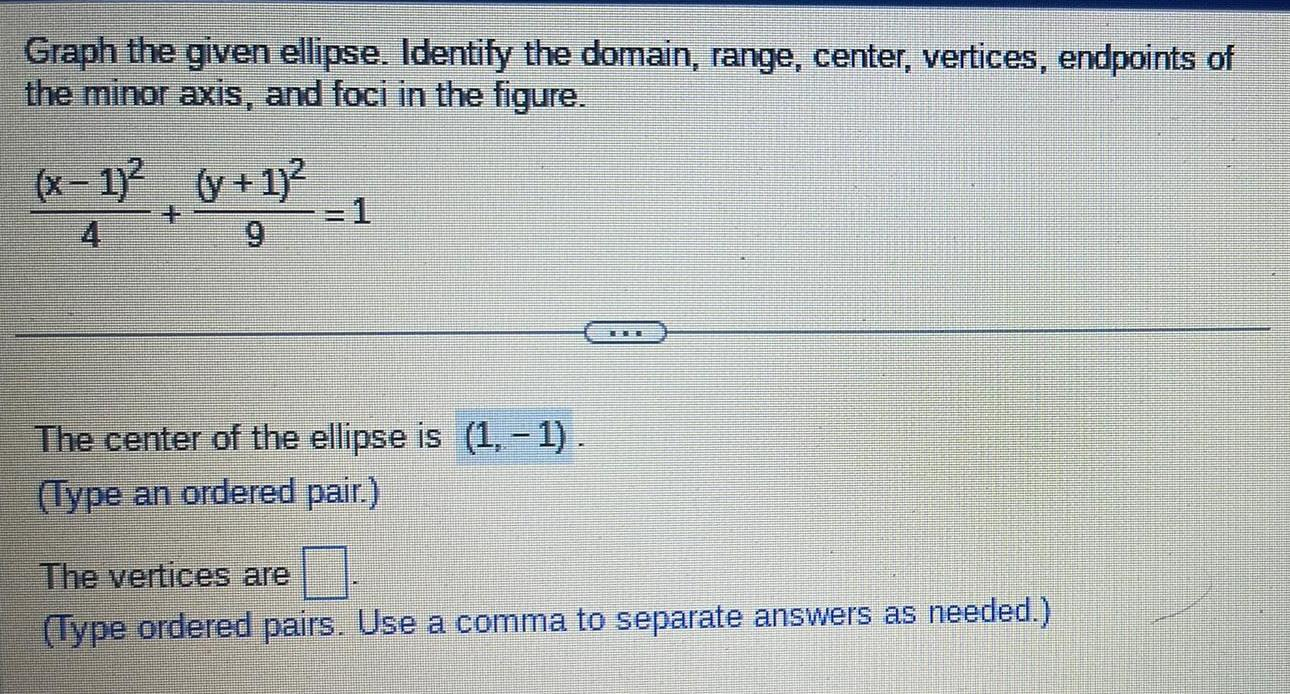Question:

# Graph the given ellipse. Identify the domain, range, center,

Last updated: 8/13/2022Graph the given ellipse. Identify the domain, range, center, vertices, endpoints of the minor axis, and foci in the figure. (x-1)²/4 + (y + 1)²/9 =1 The center of the ellipse is (1,-1). (Type an ordered pair.) The vertices are . (Type ordered pairs. Use a comma to separate answers as needed.)